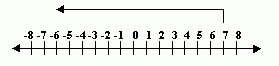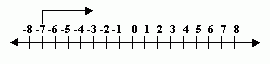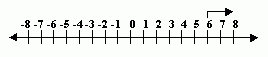Name: ___________________Date:___________________

 Email us to get an instant 20% discount on highly effective K-12 Math & English kwizNET Programs!

### MEAP Preparation - Grade 7 Mathematics1.126 Adding Integers using Number Line

 Adding two positive numbers: The operation is addition and the result is a positive number. Adding one positive and one negative number: The operation is subtraction and the result can be either positive or negative. Do not confuse the sign of the integer with the operation being performed. Adding two negative numbers: The operation is addition and the result is a negative number. Examples:Directions: Answer the following questions. Also write at least ten examples of your own.Name: ___________________Date:___________________

### MEAP Preparation - Grade 7 Mathematics1.126 Adding Integers using Number Line

 Q 1: (-5) + 8 =-332 Q 2: 7 + -13 = 7 - 13 =-667 Q 3: (-7) + 4 =03-3 Q 4: 6 + 2 =-878 Question 5: This question is available to subscribers only! Question 6: This question is available to subscribers only!

#### Subscription to kwizNET Learning System costs less than \$1 per month & offers the following benefits:

• Unrestricted access to grade appropriate lessons, quizzes, & printable worksheets
• Instant scoring of online quizzes
• Progress tracking and award certificates to keep your student motivated
• Unlimited practice with auto-generated 'WIZ MATH' quizzes